HomeBlogData ScienceData Visualization in Python: Fundamental Plots Explained [With Graphical Illustration]

# Data Visualization in Python: Fundamental Plots Explained [With Graphical Illustration]

Last updated:
12th Jun, 2023
Views
10 MinsView All## Basic Design Principles

For any aspiring or successful data scientist, being able to explain your research and analysis is a very important and useful skill to possess. This is where data visualization comes into the picture. It is vital to use this tool honestly as the audience can be very easily misinformed or deceived by poor design choices.

As data scientists, we all have certain obligations in the matter of preserving what is true.

The first is that we should be completely honest with ourselves while cleaning and summarizing the data. Data pre-processing is a very crucial step for any machine learning algorithm to work and so any dishonesty in the data will lead to drastically different results.

Another obligation is towards our target audience. There are various techniques in data visualization which are used to highlight specific sections of data and make some other pieces of data less prominent. So if we are not careful enough, the reader will not be able to explore and judge the analysis properly which can lead to doubts and a lack of trust.

Always questioning oneself is a good trait to have for data scientists. And we should always think about how to show what truly matters in an understandable as well as aesthetically pleasing way, while also remembering that context is important.

This is exactly what Alberto Cairo tries to portray in his teachings. He mentions the Five Qualities of Great Visualizations: beautiful, enlightening, functional, insightful, and truthful which are worth keeping in mind.

## How To Choose Visualization Type?

The following tips can help you choose the most suitable data visualization using Python.

• When accurate quantities of numbers must be known, the tabular format works best.
• When attempting to visualize continuous data across time, line charts work best.
• The ideal applications for bar charts are comparisons between categories.
• Pie charts work best when comparing pieces to the entire picture.
• A heat map is the easiest way to display a geographic representation of data.
• Scatter charts work well when displaying values for two variables from a dataset. They are excellent at illuminating the general relationship in a huge body of information.
• Area charts monitor changes over time for one or more groups.
• Scatter plots can illustrate and demonstrate the relationships between three variables using a bubble chart.
• A box plot displays the distribution’s shape, center, and variability.

## Some Fundamental Plots

Now that we have a basic understanding of design principles, let’s dive into some fundamental visualization techniques using the matplotlib library in python.

All the code below can be executed in a Jupyter notebook.

%matplotlib notebook

# this provides an interactive environment and sets the back end. (%matplotlib inline can also be used but it’s not interactive. This means that any further calls to plotting functions will not automatically update our original visualization.)

import matplotlib.pyplot as plt  # importing the required library module

## Point Plots

The simplest matplotlib function to plot a point is plot(). The arguments represent X and Y coordinates, then a string value that describes how the data output should be shown.

plt.figure()

plt.plot( 5, 6, ‘+’ ) # the + sign acts as a marker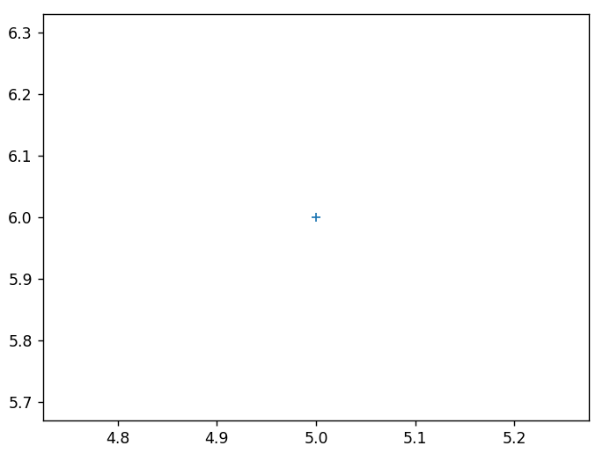## Scatterplots

A scatterplot is a two-dimensional plot. The scatter() function also takes the X value as a first argument and Y value as the second. The plot below is a diagonal line and matplotlib automatically adjusts the size of both axes. Here, the scatter plot doesn’t treat the items as a series. So, we can also give in a list of desired colors corresponding to each of the points.

import numpy as np

x = np.array( [1, 2, 3, 4, 5, 6, 7, 8] )

y = x

plt.figure()

plt.scatter( x, y )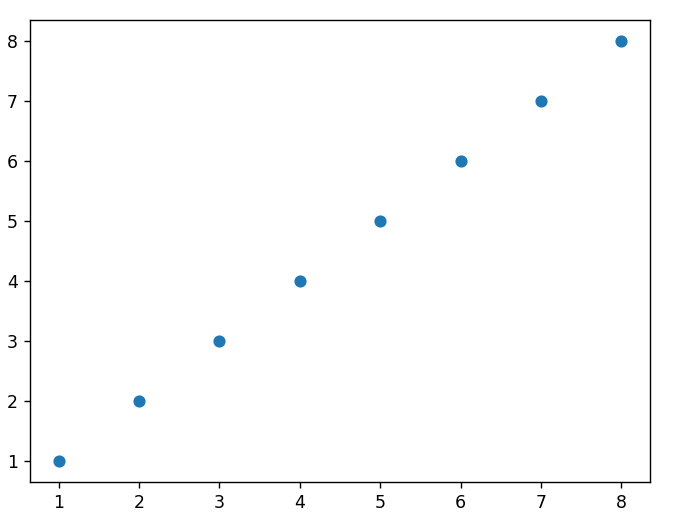## Explore our Popular Data Science Online Courses

 Executive Post Graduate Programme in Data Science from IIITB Professional Certificate Program in Data Science for Business Decision Making Master of Science in Data Science from University of Arizona Advanced Certificate Programme in Data Science from IIITB Professional Certificate Program in Data Science and Business Analytics from University of Maryland Data Science Online Courses

## Histogram

A histogram is another method of data visualization in Python. It is a graphic depiction of a frequency distribution of grouped continuous classes. In essence, a histogram shows data divided into multiple groups. It is a technique to graphically represent the distribution of numerical data. As shown in the figure below, the X-axis in a histogram displays the bin ranges, a total bill in this case, and the Y-axis displays the count.

The syntax used for the histogram:

```sns.histplot(x='totalbill', data=data, kde=True)

plt.show()```

## Heatmaps

A heatmap is a Python visualisation method that allows the visualization of a correlation matrix, time-series movements, temperature variations, and confusion matrix. You may visualize your data by using heatmaps. They can show significant correlations in your data in a variety of contexts.

The syntax used for heatmaps:

```hm = sn.heatmap(data = data)

plt.show()```

## Line Plots

A line plot is created with the plot() function and plots a number of different series of data points like a scatter plot but it connects each point series with a line.

import numpy as np

linear_data = np.array( [1, 2, 3, 4, 5, 6, 7, 8] )

squared_data = linear_data**2

plt.figure()

plt.plot( linear_data, ‘-o’, squared_data, ‘-o’)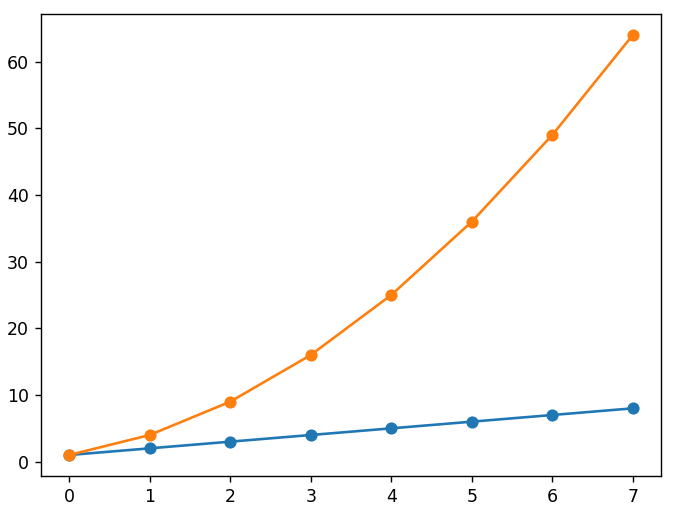To make the graph more readable, we can also add a legend which will tell us what each line represents. A suitable title for the graph and both the axes is important. Also any section of the graph can be shaded using the fill_between() function to highlight relevant regions.

## Read our popular Data Science Articles

 Data Science Career Path: A Comprehensive Career Guide Data Science Career Growth: The Future of Work is here Why is Data Science Important? 8 Ways Data Science Brings Value to the Business Relevance of Data Science for Managers The Ultimate Data Science Cheat Sheet Every Data Scientists Should Have Top 6 Reasons Why You Should Become a Data Scientist A Day in the Life of Data Scientist: What do they do? Myth Busted: Data Science doesn’t need Coding Business Intelligence vs Data Science: What are the differences?

plt.xlabel(‘X values’)

plt.ylabel(‘Y values’)

plt.title(‘Line Plots’)

plt.legend( [‘linear’, ‘squared’] )

plt.gca().fill_between( range ( len ( linear_data ) ), linear_data, squared_data, facecolor = ‘blue’, alpha = 0.25)

This is what the modified graph looks like-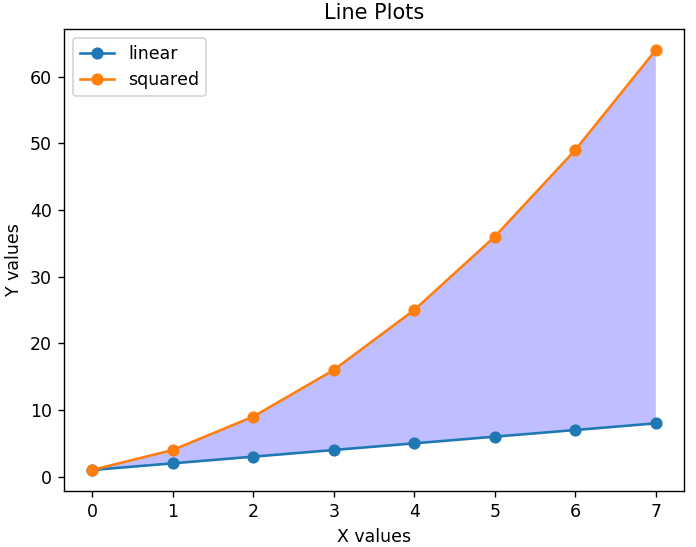## Top Data Science Skills to Learn to upskill

 SL. No Top Data Science Skills to Learn 1 Data Analysis Online Courses Inferential Statistics Online Courses 2 Hypothesis Testing Online Courses Logistic Regression Online Courses 3 Linear Regression Courses Linear Algebra for Analysis Online Courses

## Bar Charts

We can plot a bar chart by sending in arguments for the X values and the height of each bar to the bar() function. Below is a bar plot of the same linear data array we used above.

plt.figure()

x = range( len ( linear_data ))

plt.bar( x, linear_data )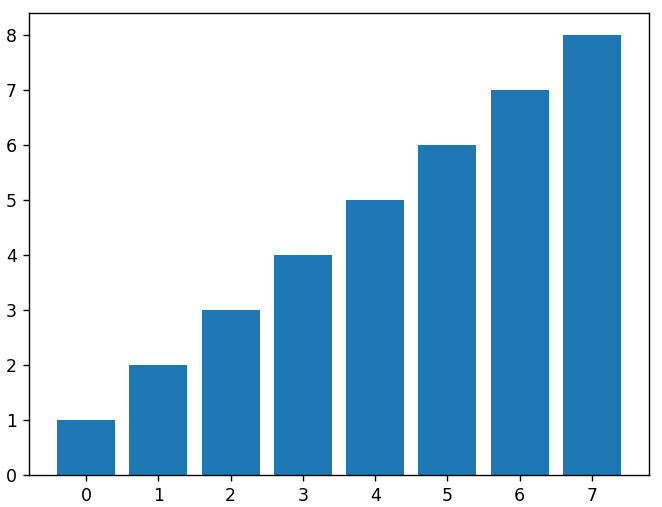# for plotting the squared data as another set of bars on the same graph, we have to adjust the new x values to make up for the first set of bars

new_x = []

for data in x:

new_x.append(data+0.3)

plt.bar(new_x, squared_data, width = 0.3, color = ‘green’)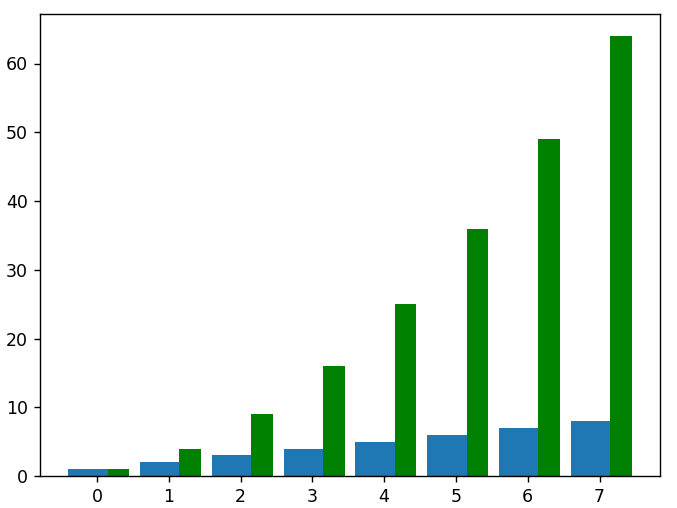# For graphs with horizontal orientation we use the barh() function

plt.figure()

x = range( len( linear_data ))

plt.barh( x, linear_data, height = 0.3, color = ‘b’)

plt.barh( x, squared_data, height = 0.3, left = linear_data, color = ‘g’)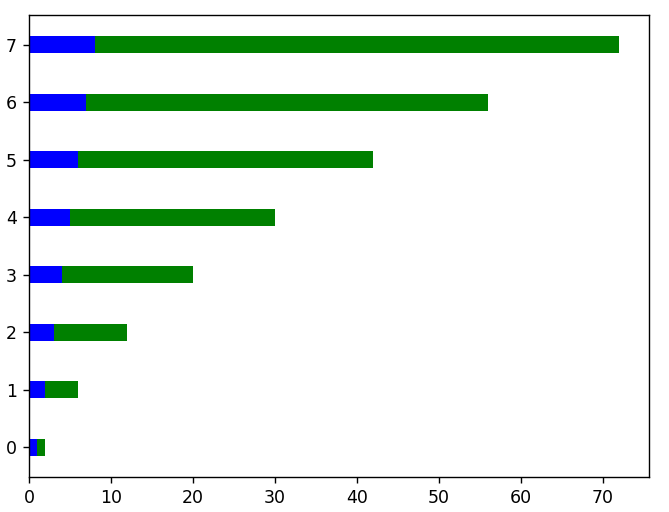#here is an example of stacking bar plots vertically

plt.figure()

x = range( len( linear_data ))

plt.bar( x, linear_data, width = 0.3, color = ‘b’)

plt.bar( x, squared_data, width = 0.3, bottom = linear_data, color = ‘g’)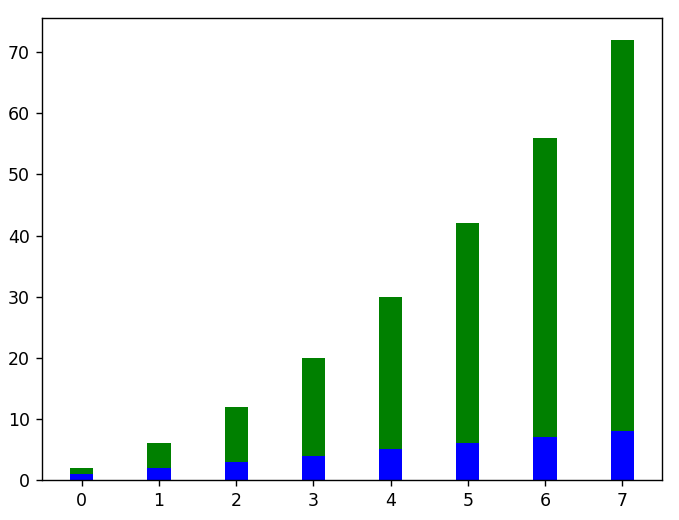Learn data science courses from the World’s top Universities. Earn Executive PG Programs, Advanced Certificate Programs, or Masters Programs to fast-track your career.

upGrad’s Exclusive Data Science Webinar for you –

In addition to the basic techniques, some advanced techniques are as follows:

• Network Visualization: It helps visualize relationships between entities like social networks, supply chain networks, and transportation networks. You can opt for NetworkX and Gephi to carry out network visualization in Python. While NetworkX is ideal for creating, manipulating, and studying complex networks, Gephi helps with network analysis.
• Geographic Visualization: It is a useful technique to display data on a map. You can depict demographic, transportation, or even environmental data. Carry out geographic visualization in Python using libraries like Basemap and Folium. Basemap for plotting 2D data on maps and Folium for creating interactive maps with Leaflet.js.
• 3D Visualization: This technique is best for representing data in a three-dimensional space. Some Python libraries, namely Matplotlib, Mayavi, and Plotly are suitable for 3D visualization.

## Conclusion

The visualization types don’t just end here. Python also has a great library called seaborn which is definitely worth exploring. Proper information visualization greatly helps increase the value of our data. Data visualization will always be the better option for gaining insights and identifying various trends and patterns rather than looking through boring tables with millions of records.

If you are curious to learn about data science, check out IIIT-B & upGrad’s PG Diploma in Data Science which is created for working professionals and offers 10+ case studies & projects, practical hands-on workshops, mentorship with industry experts, 1-on-1 with industry mentors, 400+ hours of learning and job assistance with top firms.#### Rohit Sharma

Blog Author
Rohit Sharma is the Program Director for the UpGrad-IIIT Bangalore, PG Diploma Data Analytics Program.
Get Free Consultation

Select CourseSelectBy clicking 'Submit' you Agree to

#### Data Science Skills to Master

1What are some useful Python packages for data visualization?

2. Seaborn - The Seaborn library is used for statistical representations in Python. It is developed on the top of Matplotlib and is integrated with Pandas data structures.
3. Altair - Altair is another popular Python library for data visualization. It is a declarative statistical library that allows you to create visuals with minimum possible coding.
4. Plotly - Plotly is an interactive and open-source data visualization library of Python. The visuals created by this browser-based library are supported by many platforms such as Jupyter Notebook and standalone HTML files.

2What do you know about point plots and scatter plots?

The point plots are the most basic and simplest plots for data visualization. A point plot displays the data in the form of points on a cartesian plane. The “+” shows the increase in the value while “-” shows the decrease in the value over time.
A Scatter plot on the other hand is an optimized plot where the data is visualized on a 2-D plane. It is defined using the scatter() function that takes the x-axis value as the first parameter and the y-axis value as the second parameter.

3What are the advantages of data visualization?

The following advantages show how data visualizations can become the real hero for an organization’s growth:
1. Data visualization makes it easier to interpret the raw data and understand it for further analysis.
2. After researching and analysing the data, the results can be displayed using meaningful visualizations. This makes it easier to connect with the audience and explain the results.
3. One of the most essential applications of this technique is to analyze patterns and trends to deduce predictions and potential areas of growth.
4. It also allows you to segregate the data according to customer preferences. You can also identify the areas that need more attention.

## Suggested Blogs

295133
Summary: In this Article, you will learn about 4 Types of Data Qualitative Data Type Nominal Ordinal Quantitative Data Type Discrete Continuous R